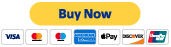#### Part 1

Discover key mathematical concepts such as expanded form and place value, comparing and ordering numbers, the relationship between fractions and decimals, and rounding numbers to various place values.

TEKS 4.2b-d, g

#### Part 5

Examine geometry and measurement concepts such as triangles, classifying 2-D shapes, measuring and drawing angles with a protractor, and relative weight.

TEKS 4.6c-d, 4.7c, 4.8a

#### Part 2

Take a look at comparing fractions, both with like and unlike denominators, how to represent fractions and decimals on a number line, and how to add and subtract both decimals and fractions.

TEKS 4.3d-e, g, 4.4a

#### Part 6

Take a look at data analysis concepts such as dot plots and frequency tables, stem-and-leaf plots, and personal financial literacy.

TEKS 4.9a-b, d

#### Part 3

Learn about patterns of multiplying by 10 and 100, 2-digit multiplication, long division, including interpreting remainders, and 2-step multiplication and division problems.

TEKS 4.4b, d, f, h

#### Part 4

Uncover operations and algebraic expressions, estimation, input-output tables, representing unknown quantities, and geometric concepts such as area, perimeter, and symmetry.

TEKS 4.4g, 4.5a-b, d, 4.6bTake the 6 hour CPE course (\$15)